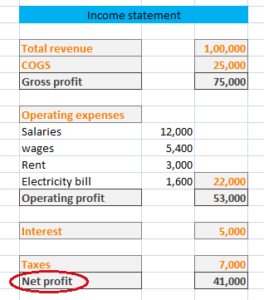## Net profit – Meaning & Formula

Net profit is the excess of a company’s net revenue (net sales) over its total expenses. For this purpose, total expenses include both direct and indirect expenses as well as interest and taxes.

If a company is liable to pay dividend to preference shareholders then the amount of dividend is also subtracted to get this figure. Here, it should be noted that the dividend paid to equity shareholders is not deducted from net sales.

Net profit is the also known as Profit after tax (PAT).

### Formula for the calculation of net profit:

Net sales – COGS* – Operating expenses – Interest and taxes – Dividend to preference shareholders (If any)

Or

Gross profit – Operating expenses – Interest and taxes – Dividend to preference shareholders (If any)

Or

Operating profit – Interest and Taxes – Dividend to preference shareholders (If any)

*Cost of goods sold (COGS) = Opening stock + Purchases – Closing stock

### Example:

From the figures provided in income statement of XYZ ltd, PAT can be calculated as:Net profit = Gross profit – Operating expenses – Interest and taxes

= 75,000 – 22,000 – 12,000* = 41,000

Or

Operating profit – (Interest and Taxes)

= 53,000 – 12,000* = 41,000

* The given figure is the sum total of Interest and Taxes.

## Net profit margin – Meaning & Formula

Net profit margin is calculated by dividing the amount of net profit by net sale. This is one of the important profitability ratios used to gauge the overall profitability of a firm. This ratio is often used to measure the efficiency of a management to derive profit out of its sale.

Net profit is the amount which is left after deducting all direct and indirect expenses from the amount of total sales.

Net profit = Operating profit – Interest and taxes

In case a company pays dividend to its shareholders then dividend paid to preference shareholders (not to equity shareholders) is also deducted to get the net profit.

### Formula for the calculation of net profit margin:

Net profit ratio = Net profit/ Net revenue

The higher degree of this ratio shows that management is effectively generating profit while incurring lower costs.

## Operating profit margin – Meaning & Formula

Operating profit margin is one of the profitability ratios used to gauge the operating profitability of a firm. This ratio represents the relationship between operating profit and net sale of a firm.

Operating profit is calculated by subtracting the amount of cost of goods sold (COGS) and operating expenses from total sales. This is the profit which includes interest and taxes amount. That’s why, it is also known as ‘Earning before interest and taxes (EBIT)’.

Operating profit margin is also known as ‘Operating margin’.

### Formula for calculation of operating profit margin:

Operating margin is calculated by dividing the amount of operating profit by net revenue (net sale).

Operating profit ratio = Operating profit/ Net sale

As operating profit is calculated by deducting the amount of operating expenses from the amount of gross profit, below formula can also be used in calculation of operating margin

Operating profit ratio = (Gross profit – Operating expenses)/ Net sales

The higher degree of this ratio shows that management is effectively generating profit while incurring lower operating costs.

## Gross profit margin – Meaning & Formula

Gross profit margin is one of the profitability ratios used to gauge the general profitability of a firm. This ratio represents the relationship between gross profit and net sale of a firm. The main usability of this ratio is to measure the effectiveness of firm to generate profit out of its sale.

Gross profit margin is also known as ‘Gross margin’.

### Formula for calculation of gross profit margin:

Gross margin is calculated by dividing the amount of gross profit by net revenue (net sale).

Gross profit ratio = Gross profit/ Net sale

Gross profit is calculated by deducting the amount of cost of goods sold from net sales. Therefore, below formula can also be used in calculation of gross profit ratio

Gross profit ratio = (Sales – COGS)/ Net sales

Cost of goods sold (COGS) = Opening stock + Purchases – Closing stock

The higher degree of this ratio shows that management is effectively generating profit while incurring lower cost of goods sold.

## Deduction – An important step of O2C process

Deduction is one of the important steps in order to cash cycle which is the complete process, every business enterprise follows.

This step comes into picture when an invoice has been raised and sent to customer which the customer does not pay in full. The personnel involved in this process then raises a new invoice with the amount so settled between customer and company.

For a business enterprise, deduction reduces total amount of sales and hence lead to lower profit. It also increases the total wastage of time for personnel involved in this process.

#### Reasons for deduction

The main reason for adjusting the amount of an invoice is settlement of trade promotion. Trade promotion is a technique used by companies to attract more retailers and partners.

Deduction is made in payment by customers as a result of poor quality of product, delay in shipment, incorrect invoice raised to customer, return of product by customer or any other reasons.